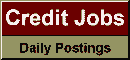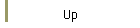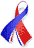DefaultRisk.com the web's biggest credit risk modeling resource.doi> search: A or B Export citation to:- HTML- Text (plain)- BibTeX- RIS- ReDIF

Are the Probabilities Right?  A first approximation to the lower bound on the number of observations required to test for default rate accuracy

by Roger M. Stein of Moody's Investors Service

May 22, 2003

Abstract: Researchers and practitioners have begun to investigate and adopt credit default models for practical applications. As a result, the issue of the accuracy of probability estimates has naturally arisen. Specifically, users of a default model that produces estimates of probabilities of default desire to know the accuracy of the probabilities produced by the model. There are a number of mechanisms for doing this, but one that has found favor due to its intuitive appeal is the estimation of goodness of fit between expected (under a particular hypothesis) and predicated default rates. While most experimenters readily acknowledge that large data sets are required to test these estimates, particularly when probabilities are small as in the case of higher credit quality borrowers, the question of how large often arises. In this short note we demonstrate, based on simple statistical relationships, how a lower bound on the size of a sample may be calculated for such experiments. It is a lower bound as it assumes no positive correlation among the data either in time or cross-sectionally, when in practice both of these assumptions are typically violated. In the presence of correlation, the bound can change considerably and we show this. That said, the bound is useful in that it can be helpful in determining when the data at hand are not sufficient to draw rigorous conclusions about the probability estimates of a model. In addition, where an experimenter has a fixed sample size, this approach provides a means for sizing the minimum difference between an estimated and an empirical default rate that should be observed in order not to conclude that the hypothesized and observed rates are statistically indistinguishable. We also discuss some of the circumstances under which the lower bound may be misleading.

Published in: Journal of Investment Management, Vol. 4, No. 2, (Q2 2006), pp. 61-71.

Books Referenced in this paper:  (what is this?)